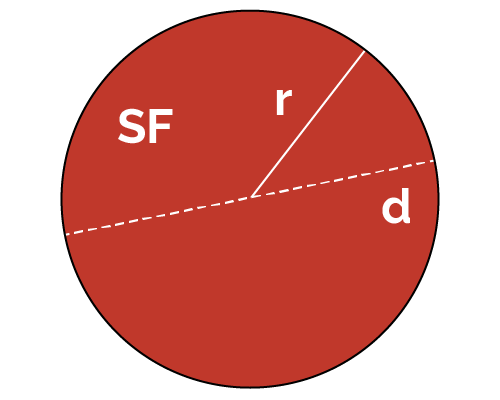# Square Footage of a Circle Calculator

Created by Luis Hoyos
Reviewed by Wojciech Sas, PhD
Last updated: Dec 16, 2022

If you want to know how to calculate the square footage of a circle, you're in the right place. This square footage of a circle calculator helps you compute the surface area in square feet, given the radius or diameter.

Keep reading to learn about the importance of square footage and how to calculate the square feet of a circle using the formula and our sq ft of a circle calculator.

## What is the square footage of a circle?

The square footage (also known as square feet, sq ft, or SF) of a circle is its area expressed in square feet instead of other area units.

Calculating a circle's square footage is relevant in designing different elements encountered in daily life, such as Ferris Wheels, enormous clocks, or maintenance hole covers. The area is an essential aspect of the design, as it's related to the cost of the materials used for building something, and sometimes you need to express it in square feet.

## How to calculate the square footage of a circle?

If you're interested in how to calculate the square feet of a circle, you have to follow a similar process to finding the area of a circle, but you must know the radius or diameter in feet. If that's not the case, you must convert the diameter to that unit, considering that 1 ft = 30.48 cm. Then, input the radius in the area of a circle formula, which turns out to be our SF formula:

SF = πr² = πd²/4

For example, to know how to find the square feet of a circle with a 1524 cm radius, follow these steps:

1. Convert 1524 cm to ft by dividing it by 30.48. The radius in cm would be: (1524/30.48) ft = 50 ft.
2. Input the radius in the SF formula:
SF = π(50 ft)² = 7854 ft².
3. Now, you know how to figure the square footage of a circle. You can check the result in our square footage calculator** by simply switching the radius units to cm and inputting 1524. Your SF should also be 7854 ft².

## Other calculators beyond sq ft of a circle calculator

Now that you know how to find the square footage of a circle with the calculator, you can look at other fascinating tools and continue to increase those geometry skills 💪

## FAQ

### How to calculate the square footage of a half-circle?

1. Remember the square footage (SF) formula of an ordinary circle, SF = πr².
2. Divide it by two, as you only want the square footage of a half-circle.
3. The result should be SF = πr²/2. That is the square footage of a half-circle for any radius.

### What is the square footage of a 16-foot circle?

201.06 ft². To find the square footage of any circle:

1. Multiply π (pi) by the diameter square: π(16 ft)² = 804.25 ft².
2. Divide that result by four:
804.25 ft²/4 = 201.06 ft².
3. That's the square footage of your circle. We use the same steps when calculating the SF of a circle formula SF = πd²/4.
Luis Hoyosft
Diameter (d)
ft
Square footage (SF)
ft²
People also viewed…

### Books vs e-Books